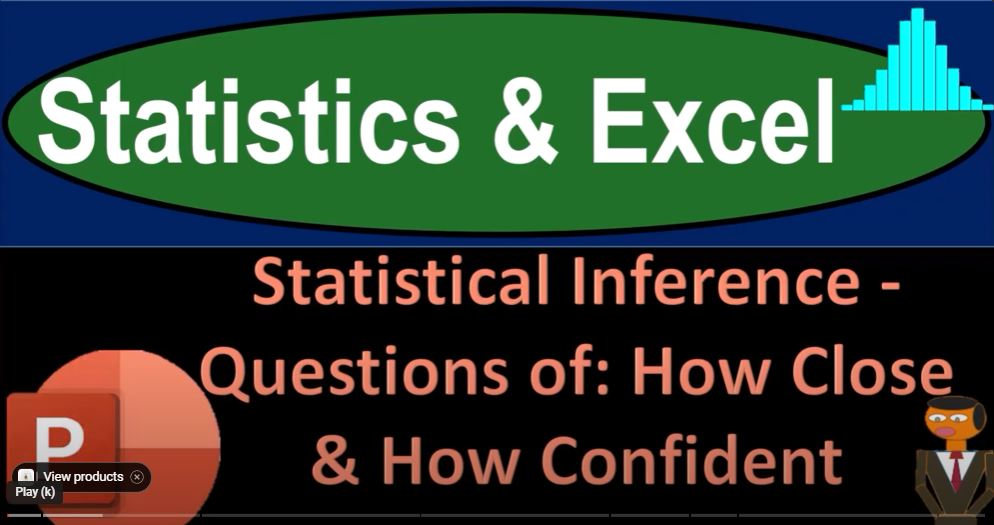## Statistical Inference – Questions of How Close & How Confident 1306 Statistics & Excel

Statistical inference is a powerful process that helps us draw meaningful conclusions about a population using data from a sample. In essence, it allows us to bridge the gap between the information we have and the broader population we want to understand. To grasp the concept of statistical inference, it’s essential to differentiate between two major categories of statistics.

Bucket 1: Known Data In the first bucket, we have all the data for the entire population. Here, we employ mathematical and pictorial tools like calculating averages, medians, quartiles, and creating histograms to understand the data.

Bucket 2: Unknown Data The second bucket, which is our focus here, involves scenarios where we don’t have data for the entire population but can gather a sample from it. Our objective is to extract meaningful insights from this sample and use them to make inferences about the entire population.

The Key Goal In statistical inference, the crucial goal is not just understanding the sample itself, but rather using it to infer characteristics of the entire population. We still analyze the sample in ways similar to the first bucket, looking at measures like averages, medians, and data spread, but our ultimate aim is predictive power for the entire population.

Approximating the Population Statistical inference aims to provide an approximate description of the larger population based on observable data from a smaller sample. In other words, the sample serves as a window through which we gain insights into the broader population.

How Close and How Confident?

The central questions in statistical inference revolve around “how close” and “how confident” we are in our data. Let’s delve into these concepts:

1. Closeness: When analyzing data, we seek to understand the middle point, shape, and spread of the sample. However, the critical aspect is determining how closely these characteristics of the sample align with those of the entire population. We want to gauge the similarity between the two.
2. Confidence: Determining confidence is a complex task. It involves assessing how confident we can be in extending the findings from our sample to the entire population. This confidence level plays a pivotal role in making informed decisions and predictions.

Practical Applications of Statistical Inference

1. Election Polling: Election predictions exemplify statistical inference in action. It’s impractical to survey every voter, so pollsters select a sample and use the results to estimate the voting behavior of the entire electorate.
2. Medical Trials: In medical trials, researchers can’t test a new drug on the entire population. Instead, they work with a sample group to infer the drug’s effectiveness for the broader population. Randomness in selecting the sample helps mitigate bias.

Randomness and Representative Samples

Random selection is fundamental in obtaining a representative sample. It ensures that each individual in the population has an equal chance of being included in the sample. While achieving perfect randomness may be challenging in practice, it’s essential to reduce bias and improve the reliability of inferences.

Estimates and Confidence Intervals

Statistical inference provides estimates of population parameters like the mean or proportion. These estimates are accompanied by confidence intervals, which represent a range of values likely to contain the true population parameter. The confidence level indicates how confident we are in the accuracy of this interval.

Probability Theory: The Backbone of Inference

Probability theory serves as the backbone of statistical inference. It equips us with the mathematical tools to quantify uncertainty and make educated predictions. It allows us to assess how likely or unlikely observed data would be under a particular statistical model.

Hypothesis Testing

Hypothesis testing is a core element of statistical inference. It involves formulating two opposing hypotheses—the null hypothesis and the alternative hypothesis. We collect data, compute a test statistic, and decide whether to reject the null hypothesis in favor of the alternative. This process enables us to make informed decisions based on sample data.

A Real-Life Example: Testing Coin Fairness

Imagine testing the fairness of a coin, where you want to determine whether it’s equally probable for the coin to land on heads or tails. By flipping the coin multiple times and analyzing the results, you can apply statistical inference to gauge the fairness of the coin.

Conclusion

Statistical inference is a vital set of tools that enables us to make informed decisions and predictions about populations based on sample data. It’s a fundamental aspect of various fields, including science, economics, medicine, business, and politics. Understanding how to apply statistical inference concepts empowers us to draw meaningful conclusions from limited data, bridging the gap between the known and the unknown.